Related Articles

# Hand-Written Digits using Topological Data Analysis

• Difficulty Level : Hard
• Last Updated : 16 Sep, 2019

Given a hand-written digit picture, we need to convert it into graph plots using point clouds.

Examples: Given a handwritten digit. We have to convert it into graph

```Input :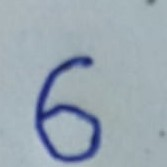Output :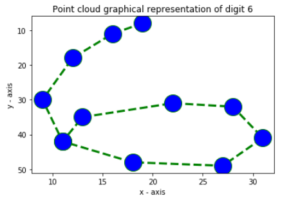```
```Input :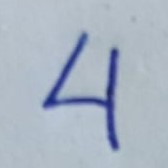Output :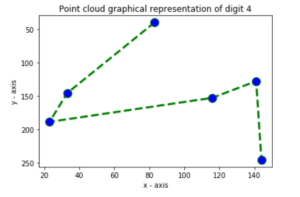```

There are some steps to follow to convert the given image to plots.

1. Binarise the image using thresholding techniques.
2. Apply component labelling of the image.
3. Using TDA Mapper, convert the image into point cloud and plot.

Step 1:
Binarisation: Binarisation means to convert the pixel image to binary image. More simply, it is to convert the image to an pixel array, that will just contain 0 and 1.

 ` ` `# Write Python3 code here``from` `PIL ``import` `Image`` ` `# read image``col ``=` `Image.``open``(``"im.pgm"``) `` ` `# conversion to gray scale``gray ``=` `col.convert(``'L'``)  `` ` `# binarization``bw ``=` `gray.point(``lambda` `x: ``0` `if` `x<``138` `else` `255``, ``'1'``)  `` ` ` ``# save it``bw.save(``"binary.png"``)``display(Image.``open``(``"binary.png"``))`

We have converted our image to binary and it looks like this-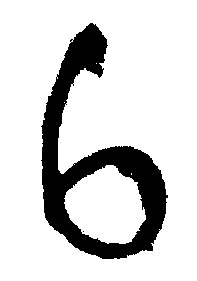Figure: Binary image

Step 2:

Component Labelling: Using component labelling we can label the picture separately along with its components. For example, we can differentiate between the holes of digit 8 and background. Here is the code for component labelling along with example.

 `# Write Python3 code here``import` `cv2``import` `numpy as np``import` `random`` ` `class` `QuickUnionUF:`` ` `    ``def` `__init__(``self``, N):``        ``self``.``id` `=` `list``(``range``(N))``        ``self``.sz ``=` `[``0``] ``*` `N`` ` `    ``@classmethod``    ``def` `fromimage(``self``, im):``        ``self``.``id` `=` `im``        ``self``.sz ``=` `[``0``] ``*` `len``(im)`` ` `    ``def` `root(``self``, i):``        ``while` `(i !``=` `self``.``id``[i]):``            ``i ``=` `self``.``id``[i]``        ``return` `i`` ` `    ``def` `getresult(``self``):``        ``result ``=` `[``self``.root(i) ``for` `i ``in` `self``.``id``]``        ``return` `result`` ` `    ``def` `connected(``self``, p, q):``        ``return` `self``.root(p) ``=``=` `self``.root(q)`` ` `    ``def` `union(``self``, p, q):``        ``i ``=` `self``.root(p)``        ``j ``=` `self``.root(q)`` ` `        ``if` `(i ``=``=` `j):``            ``return``        ``if` `(``self``.sz[i] < ``self``.sz[j]):``            ``self``.``id``[i] ``=` `j``            ``self``.sz[j] ``+``=` `self``.sz[i]``        ``else``:``            ``self``.``id``[j] ``=` `i``            ``self``.sz[j] ``+``=` `self``.sz[i]        `` ` `def` `bwlabel(im):`` ` `    ``M, N ``=` `im.shape[:``2``]``    ``qf ``=` `QuickUnionUF(M ``*` `N)``    ``for` `i ``in` `range``(M ``-` `1``):``        ``for` `j ``in` `range``(N ``-` `1``):``            ``if` `(im[i][j] ``=``=` `im[i][j ``+` `1``]):``                ``qf.union(i ``*` `N ``+` `j, i ``*` `N ``+` `j ``+` `1``)``            ``if` `(im[i ``+` `1``][j] ``=``=` `im[i][j]):``                ``qf.union(i ``*` `N ``+` `j, (i ``+` `1``) ``*` `N ``+` `j)`` ` `    ``mask ``=` `np.reshape(np.array(qf.getresult()), (M, N))``    ``values ``=` `np.unique(mask).tolist()`` ` `    ``random.seed()``    ``colors ``=` `[(random.randint(``0``, ``255``), random.randint(``0``, ``255``), ``               ``random.randint(``0``, ``255``)) ``for` `k ``in` `range``(``len``(values))]`` ` `    ``out ``=` `np.zeros((M, N, ``3``))``    ``for` `i ``in` `range``(M):``        ``for` `j ``in` `range``(N):``            ``label ``=` `values.index(mask[i][j])``            ``out[i, j] ``=` `colors[label]`` ` `    ``return` `out`` ` `im ``=` `cv2.imread(``"binary.png"``, cv2.IMREAD_GRAYSCALE)``out ``=` `bwlabel(im > ``100``)``cv2.imwrite(``"result1.png"``, out)`

Here is the output image:Figure: Component Labelled Image

As you can see, the background, the hole of 6 is differentiated by different colour.

Step 3:
Using TDA Mapper: The Mapper algorithm is a method for topological data analysis. It has large applications, a small part being, plotting maps. This package comes with Scikit-TDA of python. For installation of TDA-Mapper in PC, refer this-http://danifold.net/mapper/installation/index.html.
After installation, if we run MapperGUI.py, we will get a python application and we can input the component labelled image. After this, we will get the output image as-Figure: Graph.